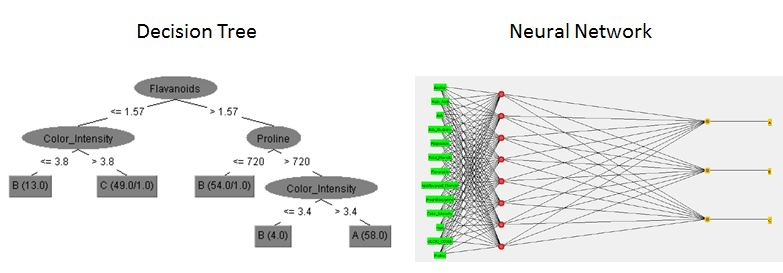October 6, 2022

# Decision Trees vs. Neural Networks

Two popular data modeling techniques are Decision Trees, also called classification trees and Neural Networks. These two data modeling techniques are very different from the way they look to the way they find relationship within variables. The neural network is an assembly of nodes, looks somewhat like the human brain. While the decision tree is an easy to follow top down approach of looking at the data.Decision Trees
Decision trees have an easy to follow natural flow. They are also easy to program for computer systems with IF, THEN, ELSE statements. We can see that the top node in the tree is the most influential piece of data that affects the response variable in the model. Because these trees are so easy to understand, they are very useful as modeling techniques and provide visual representations of the data.

Neural Networks
The neural network is not so easy to understand from the visual representation. It is very difficult to create computer systems from them, and almost impossible to create an explanation from the model. Neural networks can handle binary data better than decision trees but cannot handle categorical values.

Conclusion
From the visual representation above and the explanation of how each model handles data, one would assume that decision trees are a better model. This is an incorrect assumption because your model fit is reflective of your data set. In fact, I have seen the Neural Network achieve 99% accuracy on a data set while the decision tree model only achieved 86% accuracy on the same data set. The best fitted model is the one that most accurately fits your data.